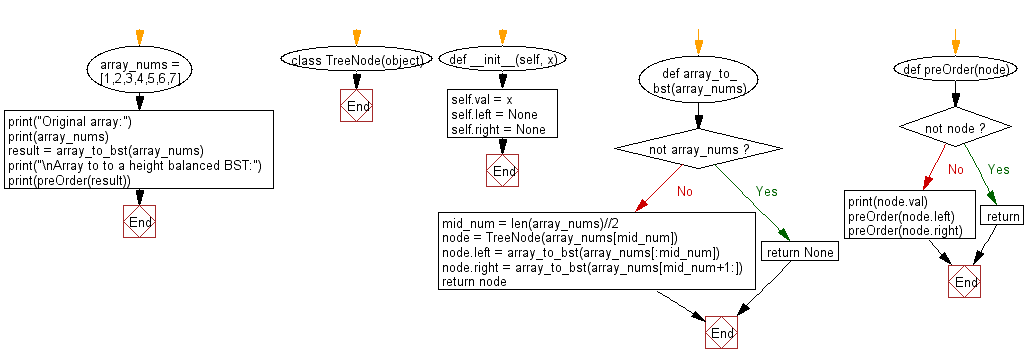﻿ Python Binary Search Tree: Convert a array to Binary Search Tree (BST) - w3resource# Python Binary Search Tree: Convert a array to Binary Search Tree (BST)

## Python Binary Search Tree: Exercise-5 with Solution

Write a Python program to convert a given array elements to a height balanced Binary Search Tree (BST).

Note: Search for a node to remove. If the node is found, delete the node.

Sample Solution:

Python Code:

``````class TreeNode(object):
def __init__(self, x):
self.val = x
self.left = None
self.right = None

def array_to_bst(array_nums):
if not array_nums:
return None
mid_num = len(array_nums)//2
node = TreeNode(array_nums[mid_num])
node.left = array_to_bst(array_nums[:mid_num])
node.right = array_to_bst(array_nums[mid_num+1:])
return node

def preOrder(node):
if not node:
return
print(node.val)
preOrder(node.left)
preOrder(node.right)

array_nums = [1,2,3,4,5,6,7]

print("Original array:")
print(array_nums)
result = array_to_bst(array_nums)
print("\nArray to a height balanced BST:")
print(preOrder(result))
```
```

Sample Output:

```Original array:
[1, 2, 3, 4, 5, 6, 7]

Array to a height balanced BST:
4
2
1
3
6
5
7
None
```

Flowchart:Python Code Editor:

What is the difficulty level of this exercise?

Test your Python skills with w3resource's quiz

﻿

## Python: Tips of the Day

Python: Use Enumerate() In for Loops

```>>> students = ('John', 'Mary',  'Mike')
>>> for i, student in enumerate(students):
...     print(f'Iteration:  {i}, Student: {student}')
...
Iteration: 0, Student: John
Iteration: 1, Student: Mary
Iteration: 2, Student: Mike
>>> for i, student in enumerate(students,  35001):
...      print(f'Student Name: {student}, Student ID #: {i}')
...
Student Name: John, Student ID #: 35001
Student Name: Mary, Student ID #: 35002
Student Name: Mike, Student ID #: 35003
```